## Problem Solving: Consecutive Letters (MIST Inter University Contest 2019)

This problem appeared in 2019 inter-university contest hosted by MIST university, Dhaka, Bangladesh. Initially, our assumption was it will be medium difficulty problem but seems like the contestants found it easier. Around 53 team out of 120 was able to solve it. Let’s see the problem statement:The time limit for this problem is $3$ seconds.

If you find the 1st query hard to understand, let me try to put it another way, you have to find the size of the largest substring which includes index $i$ and all the characters in the substring are same as $S[i]$.

Let’s see the first sample input:I give you the query “1 0”. Now you have to find the size of the largest substring which includes index $i = 0$ and contains only the character S = ‘A’. In this case the substring is S[0:1] = AA” and has size $2$ .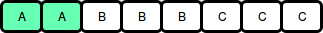Now the next query is “2 1”, so you need to replace $S$ with ‘#’ character, so the string becomes: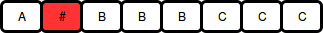After then we again query “1 0”. Now the substring is S[0:0] = A” and has size 1.Naive Solution

The first solution comes to mind is brute force. For every query of type 1, just loop from index $i$ towards left and right and see how many $S[i]$ you can find.

The time complexity for this code is O(Q*N) where N is the size of the string. As Q and N are very big, they will fail. As a problem setter, I had to be careful to make sure this code doesn’t pass. So I made some test cases where the loops for searching left and right will be very long. Those test cases have very few queries of type 2 and almost all the characters in the string are same.

Expected solution

There are several ways to solve the problem. I have solved it using a set and binary search. There is also a nice solution using a disjoint set. I will describe the solution using binary search first.

In brute force solution, you looped towards the left to find the starting point of the segment. Now I will show you how to find the $left$ point faster. Let’s forget about type 2 query for a moment.

For any given string, we can divide it by segments containing the same characters. See the following string for example:I have highlighted the starting point of each segment.

After you take the original string as input, you can easily find these points and save them, right? Let’s say we have saved them in a sorted array which looks like $[0, 3, 6, 7]$.

Now let’s say I give you a query “1 4”.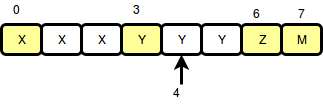Can you see that the $left$ point is the largest number in the set which is smaller than $i = 4$? In this case, it’s $3$. And how to find the $right$ point? It’s just the next number if the array, in this case, it’s $6$. So the size of the segment will be $right – left = 6 – 3 = 2$

As you have a sorted array already, you don’t need to search one by one, use the power of binary search. You can either write your own binary search or use lower_bound function available in your language and modify it.

Now how about query 2? Let’s say we put a “#” in $i = 1$. Now you can imagine this is creating a new segment from index $i = 1$.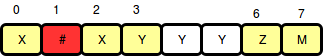Notice that not only it’s creating a segment from index $1$, it’s creating another segment from index $1 + 1 = 2$. So we insert $1$ and $2$ in our array $[0, 1, 2, 3, 6, 7]$.

Note that we never do type 1 query on a segment consisting “#”. There is no harm if you consider two consecutive “#” as two different segments. We can assume for type $1$ query, index $i$ will always create a new segment, index $i + 1$ may or may not create a new segment but we don’t need to handle it specially if you use a set.

If we use normal array to maintain the list of segment starts it will be very difficult to insert new segments as we need to keep them sorted. That’s why we will use a set. All the programming languages have some built-in set where you can insert a number in sorted way in O(LogN) complexity and also ensures uniqueness of the number.

Let’s see the code. To make our life easier, we will imagine that there is a segment starting at the very end of the string. That will save us pain of handling some special cases.

The complexity for both query of type 1 and type 2 is logN which makes the total time complexity O(Q*LogN) which is good enough to pass under 1 seconds if you use fast I/O.

Alternate solution using disjoint set

There is a nice offline solution using disjoint sets. It “offline” because you have to read all the queries first before answering them. Let’s see another example XXBBFFF, but now we will see the string as a graph: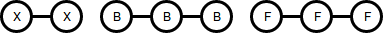All the characters converterd into nodes and there is edge between them if the characters are same. Now let’s say we have 3 queries:

Now we read all the type 2 queries first and we will erase the links with those index. In this case they are index $3$ and $5$.Now treat the connected nodes as a connected components. You can use disjoint sets to maintain the member and the size of the components. Now we have these sets $\{0, 1\}, \{2\}, \{4\}, \{5\}, \{7\}$.

Now we answer the queries from last to first! The last query is “1 5”. What is the size of the component which contains the node 6? The answer is 1.

The next query is “2 6”, now we have to unblock the 5-th node and add back the edges.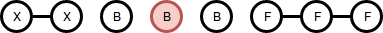Using union find algorithm, we will update the sets, now we have $\{0, 1\}, \{2\}, \{4\}, \{5, 6, 7\}$. Now we query “1 5” again, this time the answer is 3.

In this way we will climb from the end, keep unblocking the nodes and use union find to update the sets. So the type 1 query will be just finding the size of the set. In the end we have to print the queries in original order.

Union find operations have logN complexity, so in the end we have O(QlogN) time complexity. You can see a sample code here.

There is a third solution using segment tree. Code for that is significantly more complex compared to the solution described above. Also it takes much more extra memory and runs slower. During the contest we have let those solutions pass too. I am not going to describe that solution here.

That’s it for now, good wishes for your next contest.